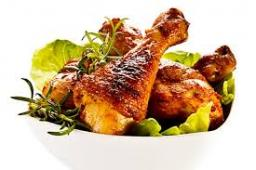# Farm2

The farm bred turkeys, geese, hens, and chickens. Everything is 400. Turkeys and geese a hen are 150. No hen has more than one chicken, but some hens have no chick. Half of them are also a quarter of the chickens. When the turkey was subtracted by 15, we got 75. How many are turkeys, geese, and hens? How many hens have no chicks?

k =  90
h =  60
s =  250

### Step-by-step explanation:

k+h+s = 400
k+h = 150
k-15=75

h+k+s = 400
h+k = 150
k = 90

Row 2 - Row 1 → Row 2
h+k+s = 400
-s = -250
k = 90

Pivot: Row 2 ↔ Row 3
h+k+s = 400
k = 90
-s = -250

s = -250/-1 = 250
k = 90/1 = 90
h = 400-k-s = 400-90-250 = 60

h = 60
k = 90
s = 250

Our linear equations calculator calculates it.Did you find an error or inaccuracy? Feel free to write us. Thank you!

Tips for related online calculators
Do you have a system of equations and looking for calculator system of linear equations?